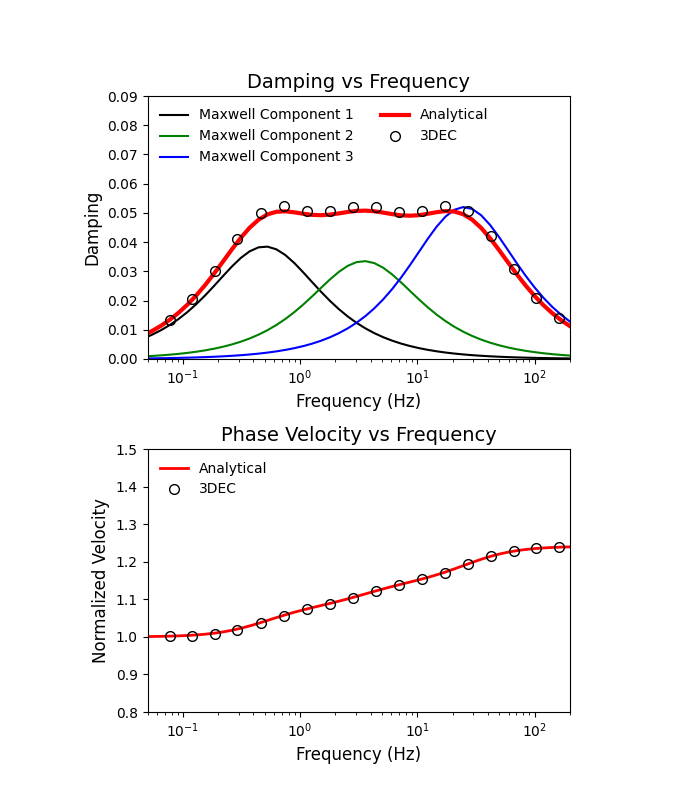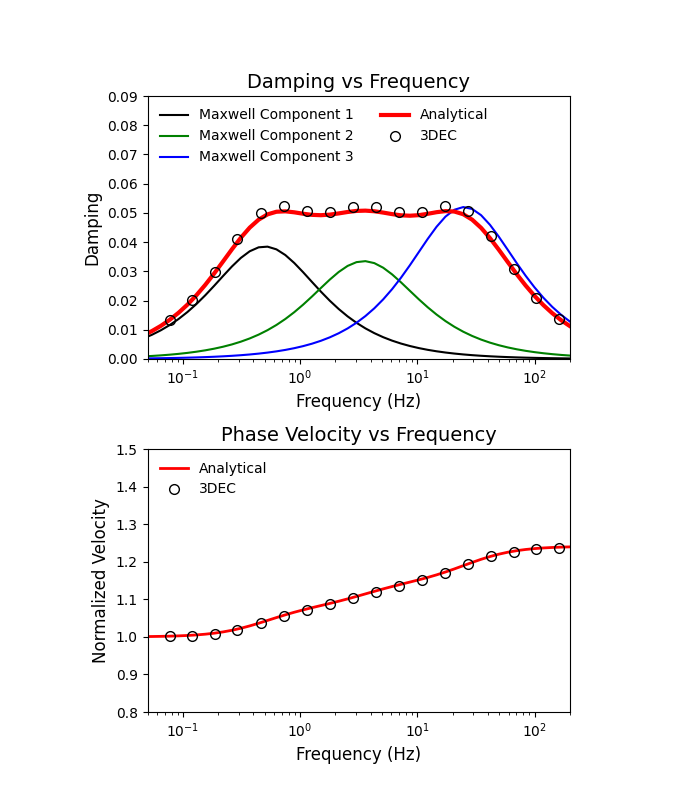# Verification problem: Maxwell damping in 3DEC

Note

To view this project in 3DEC, use the menu command Help ► Examples…. Choose “Dynamic/ Maxwell” and select “Maxwell.prj” to load. The main data files used are shown at the end of this example. The remaining data files can be found in the project.

This verification problem considers a 100-m long column. Horizontal velocities are initialized with a sine wave. Several tests are run with different stiffness properties to simulate different frequencies of vibration. Maxwell damping is applied and the damping versus frequency is compared to the analytical solution.

## Rigid Blocks

In the first test, the column is composed of 100 rigid blocks and the joint stiffnesses are altered to generate diffrent frequencies of the system. The damping versus frequency in the model compared to the analytical solution is shown in Figure 1.Figure 1: Response of rigid block model run for 21 different frequencies.

## Deformable Block

The same model is run with a single deformable block and the zone moduli are modified to produce different frequencies. The results are shown in Figure 2.Figure 2: Response of zoned block model run for 21 different frequencies.

## Deformable Blocks with Joints

In this example, 100 deformable blocks form the column and both joint and zone stiffnesses are altered to geenrate different frequency responses. The results are shown in Figure 3.Figure 3: Response of jointed and zoned block model run for 21 different frequencies.

Data Files

benchmark-rigid.dat

```; Benchmark test for Maxwell Damping

; model new
model title 'Maxwell Damping: Vibration of 1D column'
model configure dynamic
model large-strain off
fish automatic-create off
;
fish define Set_Parameters
; ------- input parameters ------------------
;global target_frequency = 1  ; frequency in hz - set in master file
global num_cycles = 3

global column_height = 100.0
global height_zones = 100

global soil_density = 2.0;
local  wave_length = 4.0*column_height
local  shear_vel = wave_length*target_frequency
local  period = 1. / target_frequency
global num_periods = num_cycles*period
global soil_shear = shear_vel*shear_vel*soil_density
global soil_poisson = 0.3
global soil_bulk = (2.*soil_shear*(1.+soil_poisson))/(3.0*(1.0-2.0*soil_poisson))

global bheight = 1.0
global jks = soil_shear * bheight
global jkn = jks * 2.0*(1.0+soil_poisson)

end
[Set_Parameters]
;
block create brick 0 1 0 1 0 [column_height]
block cut joint-set dip 0 dip-dir 0 spac [bheight] num 210 origin 0 0 0
block create brick 0 1 0 1 -1 0

block property density [soil_density]

block insitu stress -1e-6 -1e-6 -1e-6 0 0 0

block contact jmodel assign mohr
block contact property stiffness-normal [jkn] stiffness-shear [jks] friction 45 cohesion 1e20 tension 1e20

; --- Maxwell Damping Properties for 5% Damping ------------
block mech damping maxwell (0.0385 0.5) (0.0335 3.5) (0.052 25.0)
;
block fix range p-z -1 0
block fix vel-y vel-z rotation range pos-z 0 [column_height]

;
; ---- initialize velocit to quarter wave length mode ---
fish define initial_velocity
local max_vel = 1.0
;    loop foreach local p_gp block.gp.list
;        local zcord = block.gp.pos.z(p_gp)
;        local theta = (math.pi/2.)*zcord/column_height
;        block.gp.vel.x(p_gp) = max_vel*math.sin(theta)
;    end_loop
loop foreach local p_b block.list
local zcord = block.pos.z(p_b)
if zcord > 0
local theta = (math.pi/2.)*zcord/column_height
block.vel.x(p_b) = max_vel*math.sin(theta)
endif
end_loop
end
[initial_velocity]
;
model history dynamic time-total
block history displacement-x position 0 0 [column_height]
block history velocity-x position 0 0 [column_height]
block contact hist stress-shear-x pos 0 0 1

model solve time-total [num_periods]
;
history export '3' vs '1' table '10'
;
program call 'process.fis'
[data_process('rigid.csv')]
```

benchmark-zones.dat

```; Benchmark test for Maxwell Damping

; model new
model title 'Maxwell Damping: Vibration of 1D column'
model configure dynamic
model large-strain off
fish automatic-create off
;
fish define Set_Parameters
; ------- input parameters ------------------
; global target_frequency = 1  ; frequency in hz
global num_cycles = 3

global column_height = 100.0
global height_zones = 100

global soil_density = 2.0;
local  wave_length = 4.0*column_height
local  shear_vel = wave_length*target_frequency
local  period = 1. / target_frequency
global num_periods = num_cycles*period
global soil_shear = shear_vel*shear_vel*soil_density
global soil_poisson = 0.3
global soil_bulk = (2.*soil_shear*(1.+soil_poisson))/(3.0*(1.0-2.0*soil_poisson))  ;; original error - poisson, not soil_poisson
end
[Set_Parameters]
;
block create brick 0 1 0 1 0 [column_height]
block zone gen edge 1.0
block zone cmodel assign elastic
block zone property density [soil_density] shear [soil_shear] bulk [soil_bulk]
;
; --- Maxwell Damping Properties for 5% Damping ------------
block mech damping maxwell (0.0385 0.5) (0.0335 3.5) (0.052 25.0)
;
block gridpoint apply velocity-y 0.0 range p-z -1000 1000
block gridpoint apply velocity-z 0.0 range p-z -1000 1000
block gridpoint apply velocity-x 0.0 range position-z -0.1 0.1
;
; ---- initialize velocit to quarter wave length mode ---
fish define initial_velocity
local max_vel = 1.0
loop foreach local p_gp block.gp.list
local zcord = block.gp.pos.z(p_gp)
local theta = (math.pi/2.)*zcord/column_height
block.gp.vel.x(p_gp) = max_vel*math.sin(theta)
end_loop
end
[initial_velocity]
;
model history dynamic time-total
block history displacement-x position 0 0 [column_height]
block history velocity-x position 0 0 [column_height]
block history stress-xz position 0 0 0
model solve time-total [num_periods]
;
history export '3' vs '1' table '10'
;
; ------------ function time and amplitude of velocity cycle peaks ----------------
program call 'process.fis'
[data_process('zones.csv')]
```

benchmark-zones-joints.dat

```; Benchmark test for Maxwell Damping

; model new
model title 'Maxwell Damping: Vibration of 1D column'
model configure dynamic
model large-strain off
fish automatic-create off
;
fish define Set_Parameters
; ------- input parameters ------------------
; global target_frequency = 1  ; frequency in hz
global num_cycles = 3

global column_height = 100.0
global height_zones = 100

global soil_density = 2.0;
local  wave_length = 4.0*column_height
local  shear_vel = wave_length*target_frequency
local  period = 1. / target_frequency
global num_periods = num_cycles*period
global soil_shear = shear_vel*shear_vel*soil_density
global soil_poisson = 0.3
global soil_bulk = (2.*soil_shear*(1.+soil_poisson))/(3.0*(1.0-2.0*soil_poisson))  ;; original error - poisson, not soil_poisson

global bheight = 1.0
global jks = soil_shear * bheight
global jkn = jks * 2.0*(1.0+soil_poisson)

; jointed case, double soil and joint stiffnesses; keep same wave
soil_shear = 2.0*soil_shear
soil_bulk = 2.0*soil_bulk
jkn = 2.0*jkn
jks = 2.0*jks

end
[Set_Parameters]
;
block create brick 0 1 0 1 0 [column_height]
block cut joint-set dip 0 dip-dir 0 spac [bheight] num 210 origin 0 0 0
block create brick 0 1 0 1 -1 0
block zone gen edge 1.0
block zone cmodel assign elastic
block zone property density [soil_density] shear [soil_shear] bulk [soil_bulk]
block property density [soil_density]

block insitu str -1e-6 -1e-6 -1e-6 0 0 0

block contact jmodel assign mohr
block contact property stiffness-normal [jkn] stiffness-shear [jks] friction 45 cohesion 1e20 tension 1e20

; --- Maxwell Damping Properties for 5% Damping ------------
; zone dynamic damping maxwell (0.0385 0.5) (0.0335 3.5) (0.052 25.0)
block mech damping maxwell (0.0385 0.5) (0.0335 3.5) (0.052 25.0)
;
block gridpoint apply velocity-y 0.0 range p-z -1000 1000
block gridpoint apply velocity-z 0.0 range p-z -1000 1000
block gridpoint apply velocity-x 0.0 range position-z -0.1 0.1
; block fix range p-z -1 0
; block fix v-y v-z rot range p-z 0 [column_height]

;
; ---- initialize velocit to quarter wave length mode ---
fish define initial_velocity
local max_vel = 1.0
loop foreach local p_gp block.gp.list
local zcord = block.gp.pos.z(p_gp)
local theta = (math.pi/2.)*zcord/column_height
block.gp.vel.x(p_gp) = max_vel*math.sin(theta)
end_loop
;    loop foreach local p_b block.list
;        local zcord = block.pos.z(p_b)
;        if zcord > 0
;          local theta = (math.pi/2.)*zcord/column_height
;          block.vel.x(p_b) = max_vel*math.sin(theta)
;        endif
;    end_loop
end
[initial_velocity]
;
model history dynamic time-total
block history displacement-x position 0 0 [column_height]
block history velocity-x position 0 0 [column_height]
block history stress-xz position 0 0 1
block contact hist s-s-x pos 0 0 1

model solve time-total [num_periods]
;
history export '3' vs '1' table '10'
;
;
; ------------ function time and amplitude of velocity cycle peaks ----------------
program call 'process.fis'
[data_process('zones-joints.csv')]
```